1:21 AM
Keplerian Orbits

# Keplerian Orbits

Michael Fowler

## Preliminary: Polar Equations for Conic Section Curves

As we shall find, Newton's equations for particle motion in an inverse-square central force give orbits that are conic section curves. Properties of these curves are fully discussed in the accompanying "Math for Orbits" lecture, here for convenience we give the relevant polar equations for the various possibilities.

For an ellipse, with eccentricity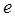and semilatus rectum (perpendicular distance from focus to curve)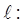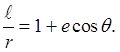Recall the eccentricityis defined by the distance from the center of the ellipse to the focus being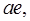where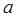is the semi-major axis, and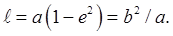For a parabola,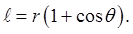For a hyperbolic orbit with an attractive inverse square force, the polar equation with origin at the center of attraction is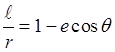where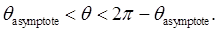(Of course, the physical path of the planet (say) is only one branch of the hyperbola.)

The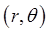origin is at the center of attraction (the Sun), geometrically this is one focus of the hyperbola, and for this attractive case it's the focus "inside" the curve.

For a hyperbolic orbit with a repulsive inverse square force (such as Rutherford scattering), the origin is the focus "outside" the curve, and to the right (in the usual representation):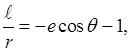with angular range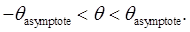## Summary

We'll begin by stating Kepler's laws, then apply Newton's Second Law to motion in a central force field. Writing the equations vectorially leads easily to the conservation laws for angular momentum and energy.

Next, we use Bernoulli's change of variable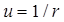to prove that the inverse-square law gives conic section orbits.

A further vectorial investigation of the equations, following Hamilton, leads naturally to an unsuspected third conserved quantity, after energy and angular momentum, the Runge Lenz vector.

Finally, we discuss the rather surprising behavior of the momentum vector as a function of time.

## Kepler's Statement of his Three Laws

1. The planets all move in elliptical orbits with the Sun at one focus.2. As a planet moves in its orbit, the line from the center of the Sun to the center of the planet sweeps out equal areas in equal times, so if the area SAB (with curved side AB) equals the area SCD, the planet takes the same time to move from A to B as it does from C to D.For my Flashlet illustrating this law, click here.

For the JavaScript version, click here.

3. The time it takes a planet to make one complete orbit around the sun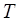(one planet year) is related to the length of the semimajor axis of the ellipse:In other words, if a table is made of the length of yearfor each planet in the Solar System, and the length of the semimajor axis of the ellipse, and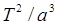is computed for each planet, the numbers are all the same.

These laws of Kepler's are precise (apart from tiny relativistic corrections, undetectable until centuries later) but they are only descriptive -- Kepler did not understand why the planets should behave in this way. Newton's great achievement was to prove that all this complicated behavior followed from one simple law of attraction.

Category: Education | Views: 342 | Added by: farrel | | Rating: 0.0/0